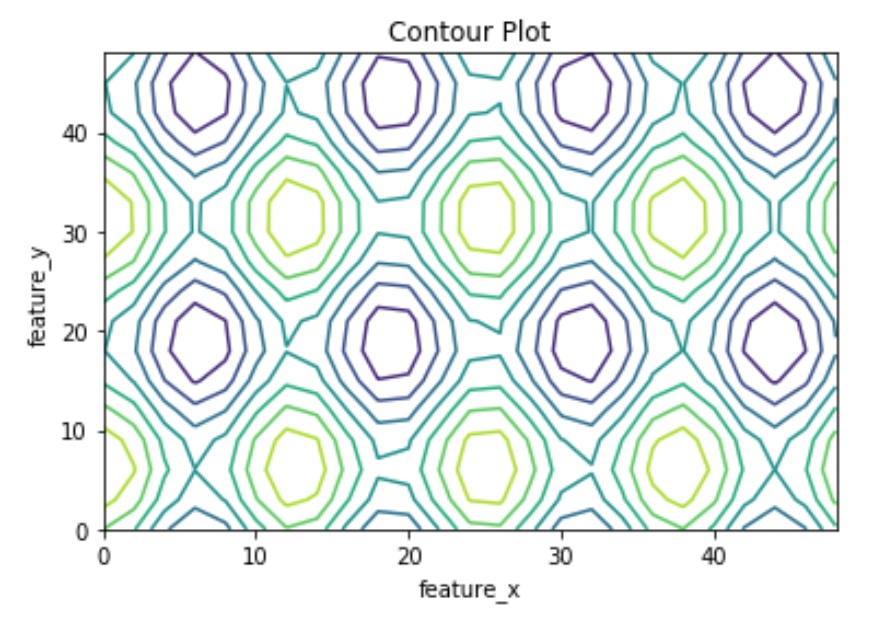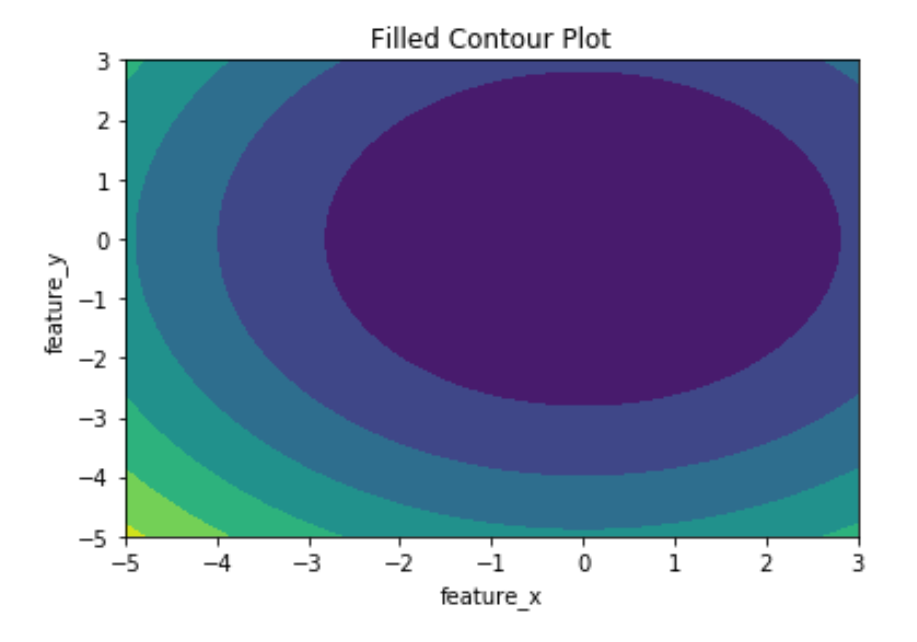# Contour Plot using Matplotlib – Python

Contour plots also called level plots are a tool for doing multivariate analysis and visualizing 3-D plots in 2-D space. If we consider X and Y as our variables we want to plot then the response Z will be plotted as slices on the X-Y plane due to which contours are sometimes referred as Z-slices or iso-response.

Contour plots are widely used to visualize density, altitudes or heights of the mountain as well as in the meteorological department. Due to such wide usage `matplotlib.pyplot` provides a method `contour` to make it easy for us to draw contour plots.

## matplotlib.pyplot.contour

The matplotlib.pyplot.contour() are usually useful when Z = f(X, Y) i.e Z changes as a function of input X and Y. A `contourf()` is also available which allows us to draw filled contours.

Syntax: matplotlib.pyplot.contour([X, Y, ] Z, [levels], **kwargs)

Parameters:
X, Y: 2-D numpy arrays with same shape as Z or 1-D arrays such that len(X)==M and len(Y)==N (where M and N are rows and columns of Z)
Z: The height values over which the contour is drawn. Shape is (M, N)
levels: Determines the number and positions of the contour lines / regions.

Below examples illustrate the `matplotlib.pyplot.contour()` function in matplotlib.pyplot:

Example #1: Plotting of Contour using `contour()` which only plots contour lines.

 `# Implementation of matplotlib function ` `import` `matplotlib.pyplot as plt ` `import` `numpy as np ` ` `  `feature_x ``=` `np.arange(``0``, ``50``, ``2``) ` `feature_y ``=` `np.arange(``0``, ``50``, ``3``) ` ` `  `# Creating 2-D grid of features ` `[X, Y] ``=` `np.meshgrid(feature_x, feature_y) ` ` `  `fig, ax ``=` `plt.subplots(``1``, ``1``) ` ` `  `Z ``=` `np.cos(X ``/` `2``) ``+` `np.sin(Y ``/` `4``) ` ` `  `# plots contour lines ` `ax.contour(X, Y, Z) ` ` `  `ax.set_title(``'Contour Plot'``) ` `ax.set_xlabel(``'feature_x'``) ` `ax.set_ylabel(``'feature_y'``) ` ` `  `plt.show() `

Output:Example #2: Plotting of contour using `contourf()` which plots filled contours.

 `# Implementation of matplotlib function ` `import` `matplotlib.pyplot as plt ` `import` `numpy as np ` ` `  `feature_x ``=` `np.linspace(``-``5.0``, ``3.0``, ``70``) ` `feature_y ``=` `np.linspace(``-``5.0``, ``3.0``, ``70``) ` ` `  `# Creating 2-D grid of features ` `[X, Y] ``=` `np.meshgrid(feature_x, feature_y) ` ` `  `fig, ax ``=` `plt.subplots(``1``, ``1``) ` ` `  `Z ``=` `X ``*``*` `2` `+` `Y ``*``*` `2` ` `  `# plots filled contour plot ` `ax.contourf(X, Y, Z) ` ` `  `ax.set_title(``'Filled Contour Plot'``) ` `ax.set_xlabel(``'feature_x'``) ` `ax.set_ylabel(``'feature_y'``) ` ` `  `plt.show() `

Output:My Personal Notes arrow_drop_upCheck out this Author's contributed articles.

If you like GeeksforGeeks and would like to contribute, you can also write an article using contribute.geeksforgeeks.org or mail your article to contribute@geeksforgeeks.org. See your article appearing on the GeeksforGeeks main page and help other Geeks.

Please Improve this article if you find anything incorrect by clicking on the "Improve Article" button below.

Article Tags :

3

Please write to us at contribute@geeksforgeeks.org to report any issue with the above content.